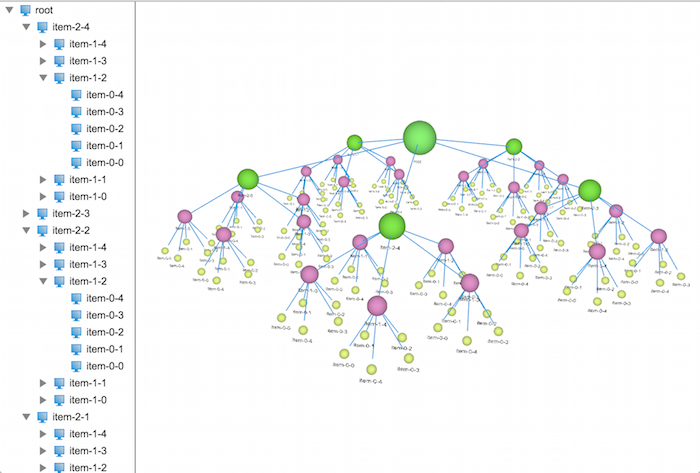## [轉載] HTML5的WebGL實現的3D和2D拓撲樹 Back

HT for Web中2D和3D应用都支持树状结构数据的展示，展现效果各异，2D上的树状结构在展现层级关系明显，但是如果数据量大的话，看起来就没那么直观，找到指定的节点比较困难，而3D上的树状结构在展现上配合HT for Web的弹力布局组件会显得比较直观，一眼望去可以把整个树状结构数据看个大概，但是在弹力布局的作用下，其层次结构看得就不是那么清晰了。所以这时候结构清晰的3D树的需求就来了，那么这个3D树具体长成啥样呢，我们来一起目睹下~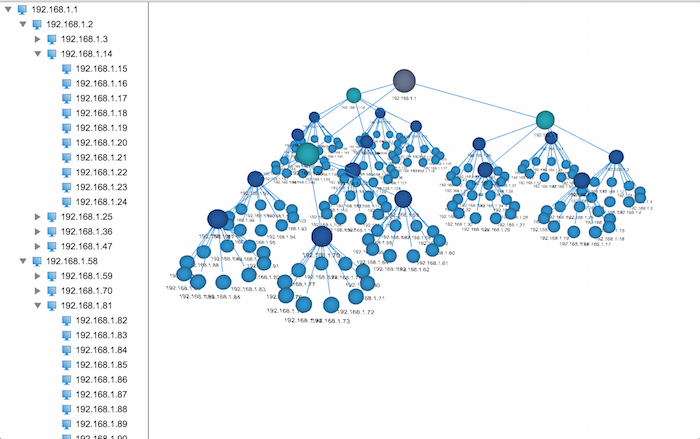### 1. 创建一个树状结构

``````/**
* 创建连线
* @param {ht.DataModel} dataModel - 数据容器
* @param {ht.Node} source - 起点
* @param {ht.Node} target - 终点
*/
function createEdge(dataModel, source, target) {
// 创建连线，链接父亲节点及孩子节点
var edge = new ht.Edge();
edge.setSource(source);
edge.setTarget(target);
}

/**
* 创建节点对象
* @param {ht.DataModel} dataModel - 数据容器
* @param {ht.Node} [parent] - 父亲节点
* @returns {ht.Node} 节点对象
*/
function createNode(dataModel, parent) {
var node = new ht.Node();
if (parent) {
// 设置父亲节点
node.setParent(parent);

createEdge(dataModel, parent, node);
}
// 添加到数据容器中
return node;
}

/**
* 创建结构树
* @param {ht.DataModel} dataModel - 数据容器
* @param {ht.Node} parent - 父亲节点
* @param {Number} level - 深度
* @param {Array} count - 每层节点个数
* @param {function(ht.Node, Number, Number)} callback - 回调函数(节点对象，节点对应的层级，节点在层级中的编号)
*/
function createTreeNodes(dataModel, parent, level, count, callback) {
level--;
var num = (typeof count === 'number' ? count : count[level]);

while (num--) {
var node = createNode(dataModel, parent);
// 调用回调函数，用户可以在回调里面设置节点相关属性
callback(node, level, num);
if (level === 0) continue;
// 递归调用创建孩子节点
createTreeNodes(dataModel, node, level, count, callback);
}
}
``````

### 2. 在2D拓扑下模拟3D树状结构每层的半径计算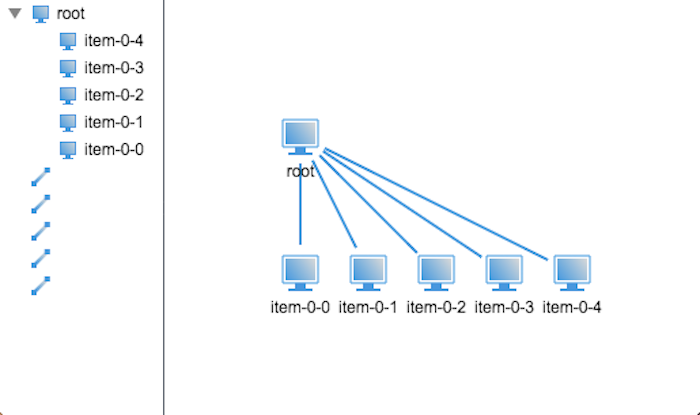r = b / 2 / sin(a / 2);

``````/**
* 布局树
* @param {ht.Node} root - 根节点
* @param {Number} [minR] - 末端节点的最小半径
*/
function layout(root, minR) {
// 设置默认半径
minR = (minR == null ? 25 : minR);
// 获取到所有的孩子节点对象数组
var children = root.getChildren().toArray();
// 获取孩子节点个数
var len = children.length;
// 计算张角
var degree = Math.PI * 2 / len;
// 根据三角函数计算绕父亲节点的半径
var sin = Math.sin(degree / 2),
r = minR / sin;
// 获取父亲节点的位置坐标
var rootPosition = root.p();

children.forEach(function(child, index) {
// 根据三角函数计算每个节点相对于父亲节点的偏移量
var s = Math.sin(degree * index),
c = Math.cos(degree * index),
x = s * r,
y = c * r;

// 设置孩子节点的位置坐标
child.p(x + rootPosition.x, y + rootPosition.y);
});
}
``````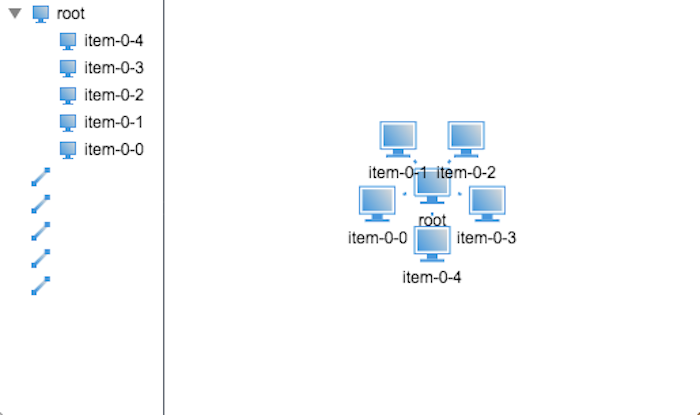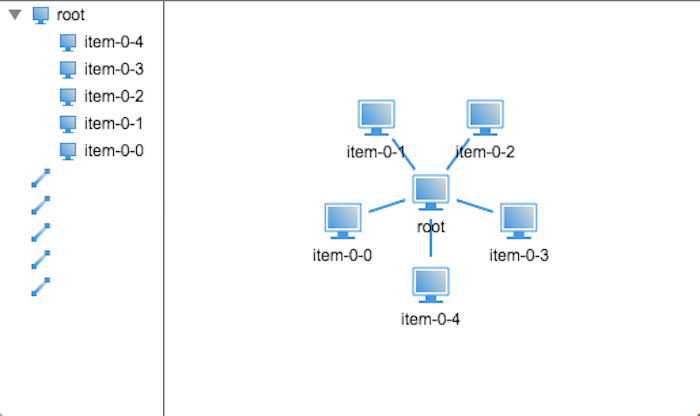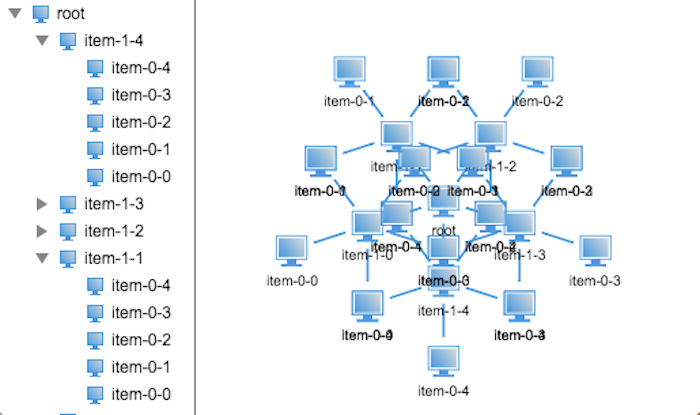``````/**
* 就按节点领域半径
* @param {ht.Node} root - 根节点对象
* @param {Number} minR - 最小半径
*/
minR = (minR == null ? 25 : minR);

// 若果是末端节点，则设置其半径为最小半径
if (!root.hasChildren()) {
return;
}

// 遍历孩子节点递归计算半径
var children = root.getChildren();
children.each(function(child) {
});

var child0 = root.getChildAt(0);
// 获取孩子节点半径

// 计算子节点的1/2张角
var degree = Math.PI / children.size();
// 计算父亲节点的半径

// 设置父亲节点的半径及其孩子节点的布局张角
root.a('degree', degree * 2);
}
``````

OK，半径的计算解决了，那么接下来就该解决布局问题了，布局树状结构数据需要明确：孩子节点的坐标位置取决于其父亲节点的坐标位置，因此布局的递归方式和计算半径的递归方式不同，我们需要先布局父亲节点再递归布局孩子节点，具体看看代码吧：

``````/**
* 布局树
* @param {ht.Node} root - 根节点
*/
function layout(root) {
// 获取到所有的孩子节点对象数组
var children = root.getChildren().toArray();
// 获取孩子节点个数
var len = children.length;
// 计算张角
var degree = root.a('degree');
// 根据三角函数计算绕父亲节点的半径
// 获取父亲节点的位置坐标
var rootPosition = root.p();

children.forEach(function(child, index) {
// 根据三角函数计算每个节点相对于父亲节点的偏移量
var s = Math.sin(degree * index),
c = Math.cos(degree * index),
x = s * r,
y = c * r;

// 设置孩子节点的位置坐标
child.p(x + rootPosition.x, y + rootPosition.y);

// 递归调用布局孩子节点
layout(child);
});
}
``````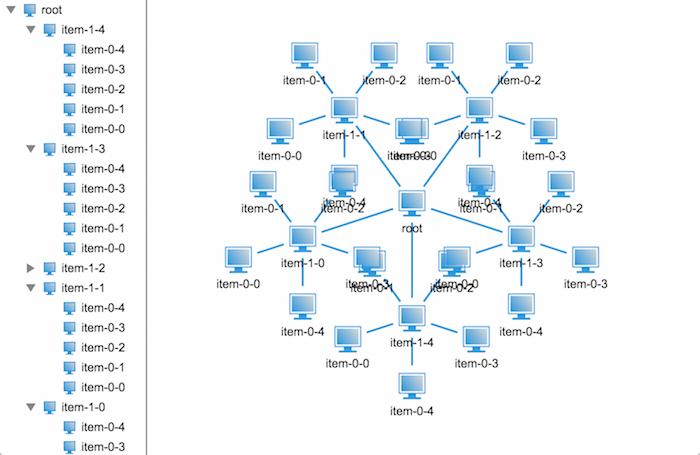``````/**
* 就按节点领域半径
* @param {ht.Node} root - 根节点对象
* @param {Number} minR - 最小半径
*/
/** ... */

var child0 = root.getChildAt(0);
// 获取孩子节点半径

var child00 = child0.getChildAt(0);
// 半径加上孙子节点半径，避免节点重叠

/** ... */
}
``````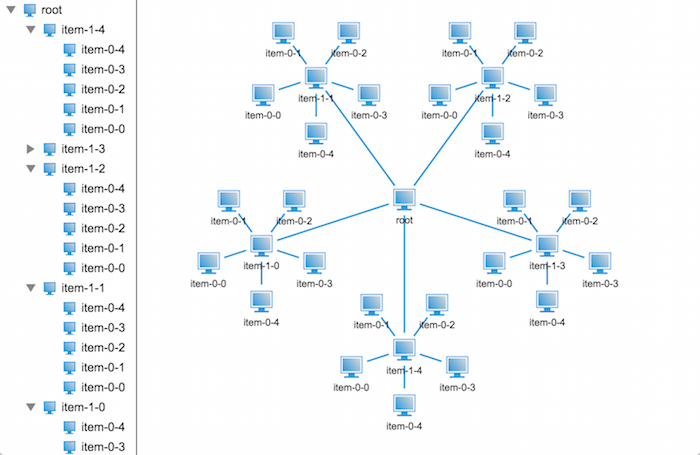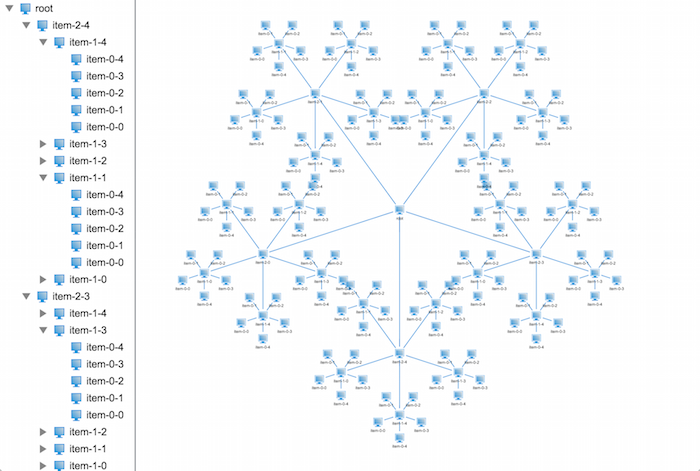OK，那我们来总结下，节点的领域半径是其下每层节点的布局半径之和，而布局半径需要根据其孩子节点个数及其领域半径共同决定。

``````/**
* 就按节点领域半径及布局半径
* @param {ht.Node} root - 根节点对象
* @param {Number} minR - 最小半径
*/
minR = (minR == null ? 25 : minR);

// 若果是末端节点，则设置其布局半径及领域半径为最小半径
if (!root.hasChildren()) {
return;
}

// 遍历孩子节点递归计算半径
var children = root.getChildren();
children.each(function(child) {
});

var child0 = root.getChildAt(0);
// 获取孩子节点半径

// 计算子节点的1/2张角
var degree = Math.PI / children.size();
// 计算父亲节点的布局半径

// 缓存父亲节点的布局半径
// 缓存父亲节点的领域半径
// 缓存其孩子节点的布局张角
root.a('degree', degree * 2);
}
``````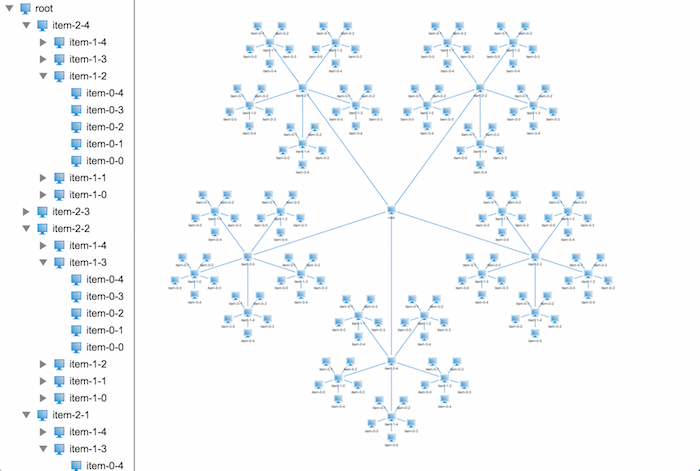### 3. 加入z轴坐标，呈现3D下的树状结构

3D拓扑上面布局无非就是多加了一个坐标系，而且这个坐标系只是控制节点的高度而已，并不会影响到节点之间的重叠，所以接下来我们来改造下我们的程序，让其能够在3D上正常布局。

``````/**
* 布局树
* @param {ht.Node} root - 根节点
*/
function layout(root) {
// 获取到所有的孩子节点对象数组
var children = root.getChildren().toArray();
// 获取孩子节点个数
var len = children.length;
// 计算张角
var degree = root.a('degree');
// 根据三角函数计算绕父亲节点的半径
// 获取父亲节点的位置坐标
var rootPosition = root.p3();

children.forEach(function(child, index) {
// 根据三角函数计算每个节点相对于父亲节点的偏移量
var s = Math.sin(degree * index),
c = Math.cos(degree * index),
x = s * r,
z = c * r;

// 设置孩子节点的位置坐标
child.p3(x + rootPosition, rootPosition - 100, z + rootPosition);

// 递归调用布局孩子节点
layout(child);
});
}
``````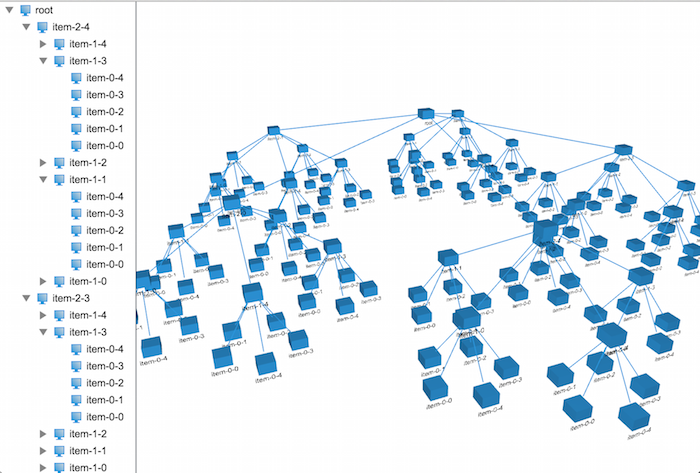``````var level = 4,
size = (level + 1) * 20;

var root = createNode(dataModel);
root.setName('root');
root.p(100, 100);

root.s('shape3d', 'sphere');
root.s('shape3d.color', randomColor());
root.s3(size, size, size);

var colors = {},
sizes = {};
createTreeNodes(dataModel, root, level - 1, 5, function(data, level, num) {
if (!colors[level]) {
colors[level] = randomColor();
sizes[level] = (level + 1) * 20;
}

size = sizes[level];

data.setName('item-' + level + '-' + num);
// 设置节点形状为球形
data.s('shape3d', 'sphere');
data.s('shape3d.color', colors[level]);
data.s3(size, size, size);
});
``````